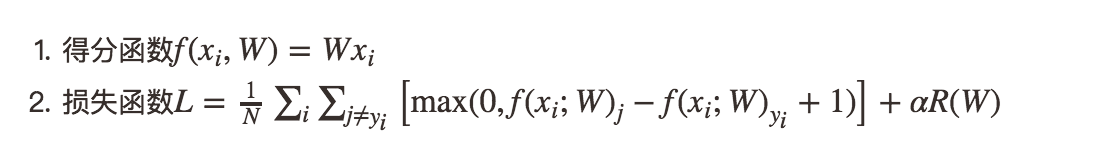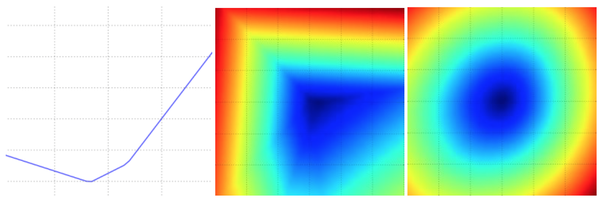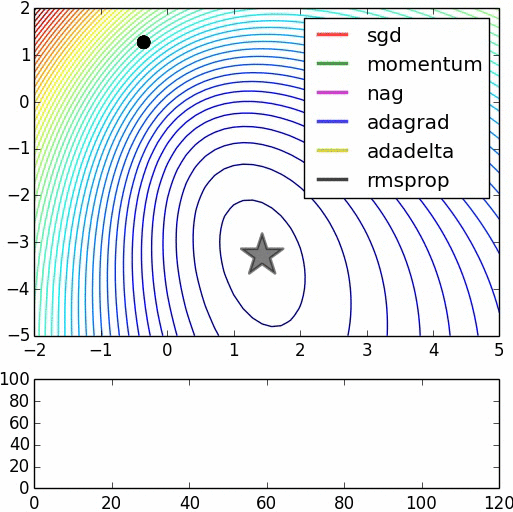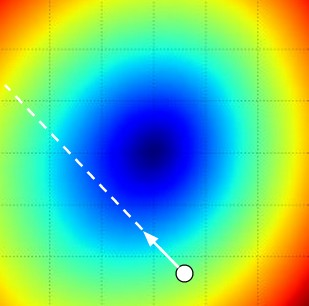# 计算机视觉之最优化与随机梯度下降

Posted by jjx on February 28, 2017

• 用于把原始像素信息映射到不同类别得分的得分函数/score function
• 用于评估参数W效果(评估该参数下每类得分和实际得分的吻合度)的损失函数/loss function#### 最优化

# 假设 X_train 是训练集 (例如. 3073 x 50,000)
# 假设 Y_train 是类别结果 (例如. 1D array of 50,000)

bestloss = float("inf") # 初始化一个最大的float值
for num in xrange(1000):
W = np.random.randn(10, 3073) * 0.0001 # 随机生成一组参数
loss = L(X_train, Y_train, W) # 计算损失函数
if loss < bestloss: # 比对已搜寻中最好的结果
bestloss = loss
bestW = W
print 'in attempt %d the loss was %f, best %f' % (num, loss, bestloss)

# prints:
# in attempt 0 the loss was 9.401632, best 9.401632
# in attempt 1 the loss was 8.959668, best 8.959668
# in attempt 2 the loss was 9.044034, best 8.959668
# in attempt 3 the loss was 9.278948, best 8.959668
# in attempt 4 the loss was 8.857370, best 8.857370
# in attempt 5 the loss was 8.943151, best 8.857370
# in attempt 6 the loss was 8.605604, best 8.605604
# ... (trunctated: continues for 1000 lines)


# 假定 X_test 为 [3073 x 10000], Y_test 为 [10000 x 1]
scores = Wbest.dot(Xte_cols) # 10 x 10000, 计算类别得分
# 找到最高得分作为结果
Yte_predict = np.argmax(scores, axis = 0)
# 计算准确度
np.mean(Yte_predict == Yte)
# 返回 0.1555


W = np.random.randn(10, 3073) * 0.001 # 初始化权重矩阵W
bestloss = float("inf")
for i in xrange(1000):
step_size = 0.0001
Wtry = W + np.random.randn(10, 3073) * step_size
loss = L(Xtr_cols, Ytr, Wtry)
if loss < bestloss:
W = Wtry
bestloss = loss
print 'iter %d loss is %f' % (i, bestloss)


$\frac{df(x)}{dx}=\lim_{h\to 0}\frac{f(x+h)-f(x)}{h}$#### 计算梯度

def eval_numerical_gradient(f, x):
"""
一个最基本的计算x点上f的梯度的算法
- f 为参数为x的函数
- x 是一个numpy的vector
"""

fx = f(x) # 计算原始点上函数值
h = 0.00001

# 对x的每个维度都计算一遍
while not it.finished:

# 计算x+h处的函数值
ix = it.multi_index
old_value = x[ix]
x[ix] = old_value + h # 加h
fxh = f(x) # 计算f(x + h)
x[ix] = old_value # 存储之前的函数值

# 计算偏导数
grad[ix] = (fxh - fx) / h # 斜率
it.iternext() # 开始下一个维度上的偏导计算



def CIFAR10_loss_fun(W):
return L(X_train, Y_train, W)

W = np.random.rand(10, 3073) * 0.001 # 随机权重向量
df = eval_numerical_gradient(CIFAR10_loss_fun, W) # 计算梯度


loss_original = CIFAR10_loss_fun(W) # 原始点上的损失
print 'original loss: %f' % (loss_original, )

# 多大步伐迈进好呢？我们选一些步长试试
step_size = 10 ** step_size_log
W_new = W - step_size * df # 新的权重
loss_new = CIFAR10_loss_fun(W_new)
print 'for step size %f new loss: %f' % (step_size, loss_new)

# 输出:
# original loss: 2.200718
# for step size 1.000000e-10 new loss: 2.200652
# for step size 1.000000e-09 new loss: 2.200057
# for step size 1.000000e-08 new loss: 2.194116
# for step size 1.000000e-07 new loss: 2.135493
# for step size 1.000000e-06 new loss: 1.647802
# for step size 1.000000e-05 new loss: 2.844355
# for step size 1.000000e-04 new loss: 25.558142
# for step size 1.000000e-03 new loss: 254.086573
# for step size 1.000000e-02 new loss: 2539.370888
# for step size 1.000000e-01 new loss: 25392.214036while True:
weights += - step_size * weights_grad # 梯度下降更新参数


while True:
data_batch = sample_training_data(data, 256) # 抽样256个样本作为一个batch
weights += - step_size * weights_grad # 参数更新


• 把损失函数在各参数上的取值，想象成我们所在山峰的高度。那么我们要最小化损失函数，实际上就是『要想办法下山』。
• 我们采取的下山策略是，一次迈一小步，只要每次都往下走了，那么最后就会到山底。
• 梯度对应函数变化最快的方向，负梯度的方向就是我们下山，环顾四周之后，发现最陡的下山路方向。
• 我们的步长(也叫学习率)，会影响我们的收敛速度(下山速度)如果步伐特别特别大，甚至可能跃过最低点，跑到另外一个高值位置了。
• 我们用mini-batch的方式，用一小部分的样本子集，计算和更新参数，减少计算量，加快收敛速度。